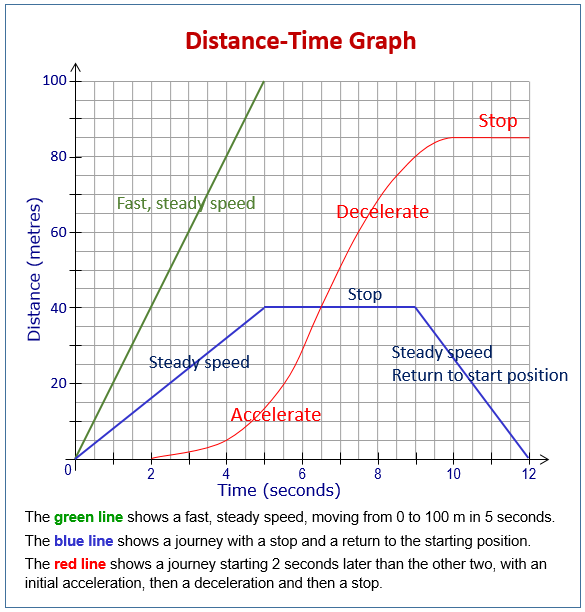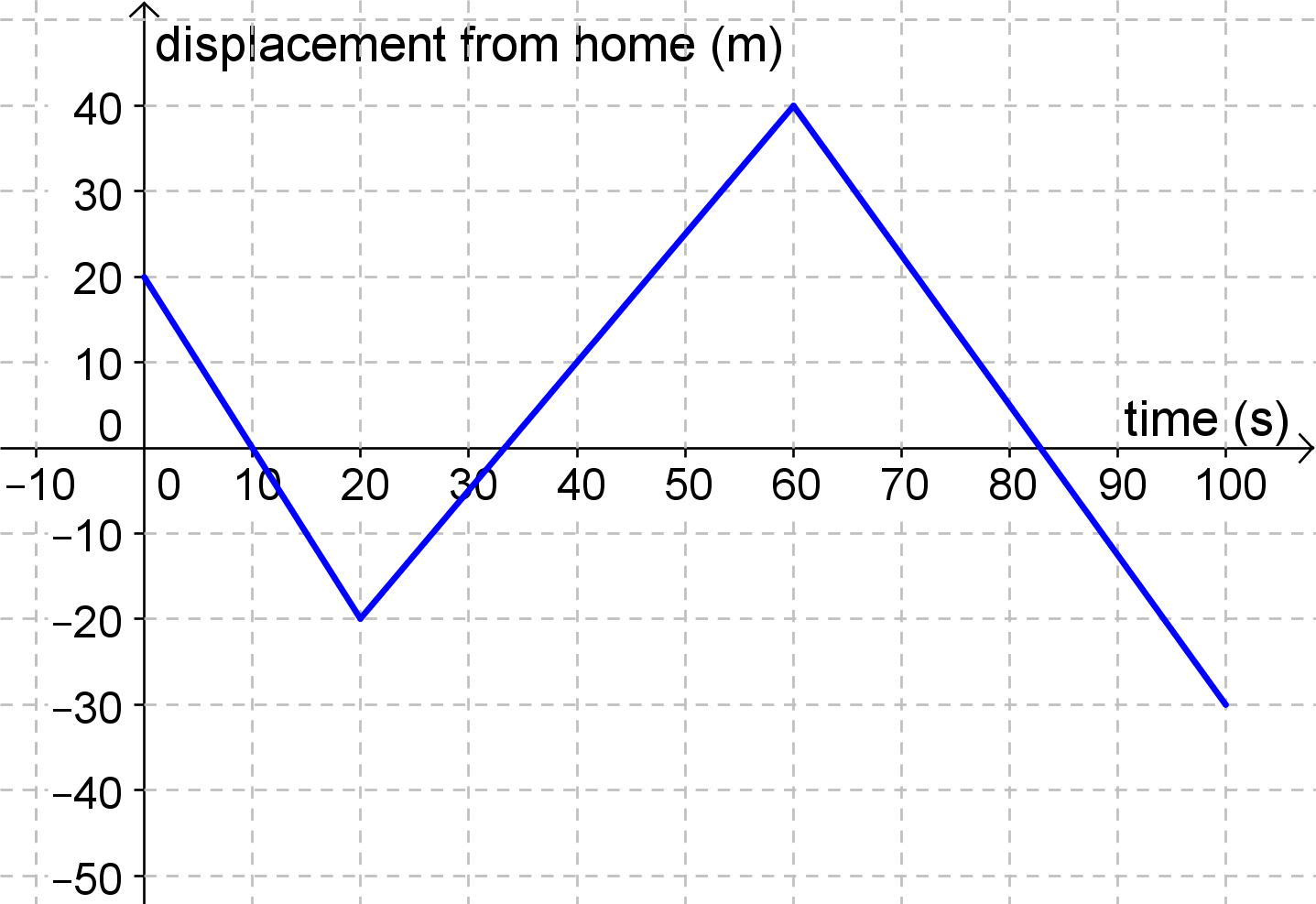# Science: Distance and Displacement

• Distance: the measurement of the length between two points
• Displacement: the CHANGE in an object’s position (straight line between start and end points)
• NOTE: An object can travel a large distance and still have zero displacement
• Distance is SCALAR (e.g. 10km) while Displacement is a VECTOR (e.g. 10km South)
• For example: If you run a 5km on a circular course, your distance traveled is 5 kilometers, regardless of where you started and finished.
• However, your displacement is ZERO if you finish where you started.

## Distance-Over-Time Graphs• NOTE: in a distance-over-time graph, Distance must ALWAYS be on the Y-Axis, while Time must ALWAYS be on the X-Axis
• If the graph is increasing, the object is IN MOTION
• If the graph is flat-lining, the object has STOPPED
• Distance-over-Time graphs CANNOT DECREASE!!! Only Displacement Graphs can decrease.
• Distance-Over-Time graphs must always be above the x-axis.

## Displacement Graphs• NOTE: in a distance-over-time graph, Distance must ALWAYS be on the Y-Axis, while Time must ALWAYS be on the X-Axis
• If the graph is flat-lining, the object has STOPPED
• If the graph is increasing, the object is moving further from the origin
• If the graph is decreasing, the object is moving closer to the origin
• If the graph intersects the x-axis, the object is at its starting point.
Mastodon